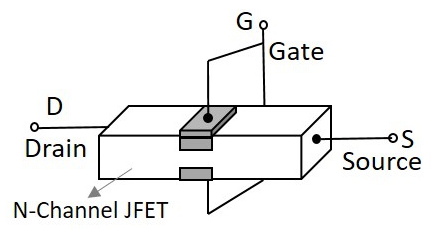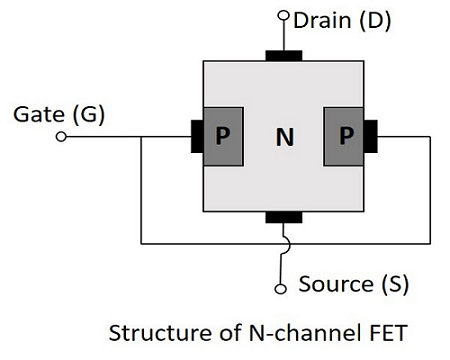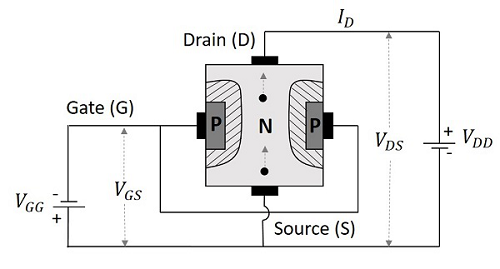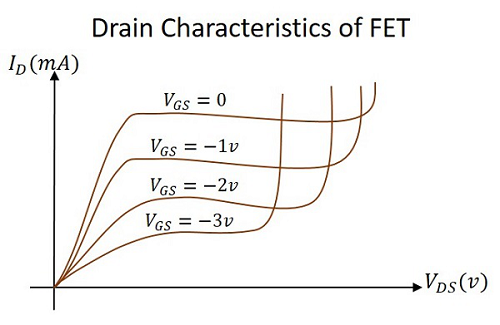## FET

The most common type of insulated gate FET which is used in many different types of electronic circuits is called the Metal Oxide Semiconductor Field Effect Transistor or MOSFET for short. ... Depletion Type – the transistor requires the Gate-Source voltage, ( VGS ) to switch the device “OFF”.

The JFET is abbreviated as Junction Field Effect Transistor. JFET is just like a normal FET. The types of JFET are n-channel FET and P-channel FET. A p-type material is added to the n-type substrate in n-channel FET, whereas an n-type material is added to the ptype substrate in p-channel FET. Hence it is enough to discuss one type of FET to understand both.

## N-Channel FET

The N-channel FET is the mostly used Field Effect Transistor. For the fabrication of Nchannel FET, a narrow bar of N-type semiconductor is taken on which P-type material is formed by diffusion on the opposite sides. These two sides are joined to draw a single connection for gate terminal. This can be understood from the following figure.These two gate depositions (p-type materials) form two PN diodes. The area between gates is called as a channel. The majority carriers pass through this channel. Hence the cross sectional form of the FET is understood as the following figure.

hmic contacts are made at the two ends of the n-type semiconductor bar, which form the source and the drain. The source and
the drain terminals may be interchanged.

## Operation of N-channel FET

Before going into the operation of the FET one should understand how the depletion layers are formed. For this, let us suppose that the voltage at gate terminal say VGG is reverse biased while the voltage at drain terminal say VDDis not applied. Let this be the case 1.

• In case 1, When VGG is reverse biased and VDD is not applied, the depletion regions between P and N layers tend to expand. This happens as the negative voltage applied, attracts the holes from the p-type layer towards the gate terminal.
• In case 2, When VDD is applied (positive terminal to drain and negative terminal to source) and VGG is not applied, the electrons flow from source to drain which constitute the drain current ID.

Let us now consider the following figure, to understand what happens when both the supplies are given.### Depletion Mode of Operation

As the width of depletion layer plays an important role in the operation of FET, the name depletion mode of operation implies. We have another mode called enhancement mode of operation, which will be discussed in the operation of MOSFETs. But JFETs have only depletion mode of operation.

Let us consider that there is no potential applied between gate and source terminals and a potential VDD is applied between drain and source. Now, a current ID flows from drain to source terminal, at its maximum as the channel width is more. Let the voltage applied between gate and source terminal VGG is reverse biased. This increases the depletion width, as discussed above. As the layers grow, the cross-section of the channel decreases and hence the drain current ID also decreases.